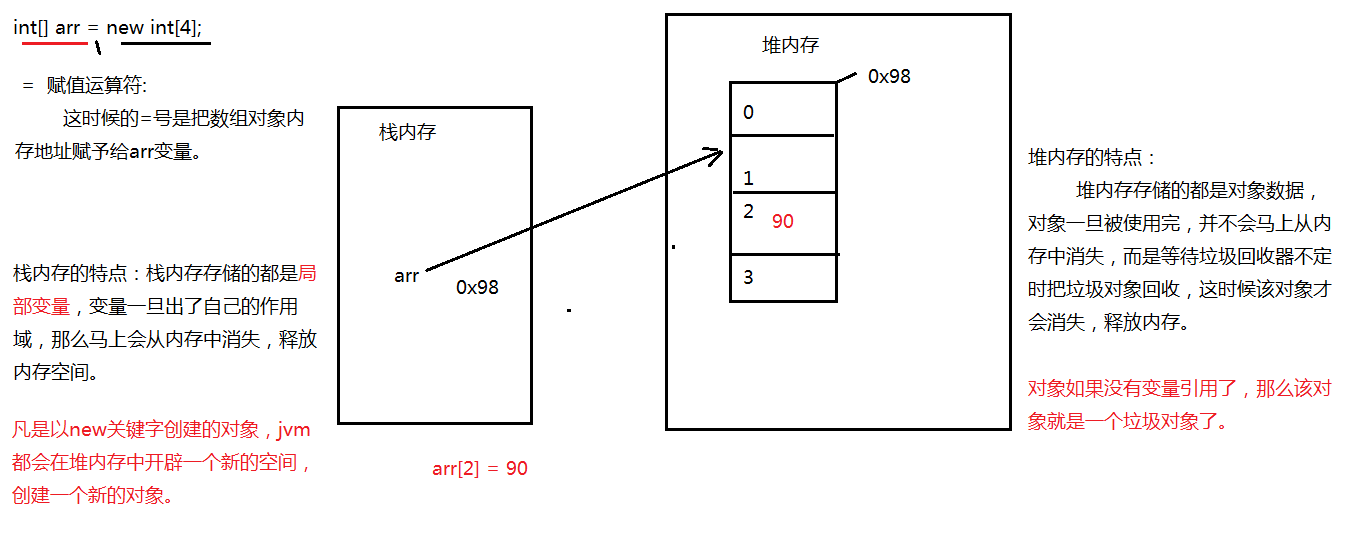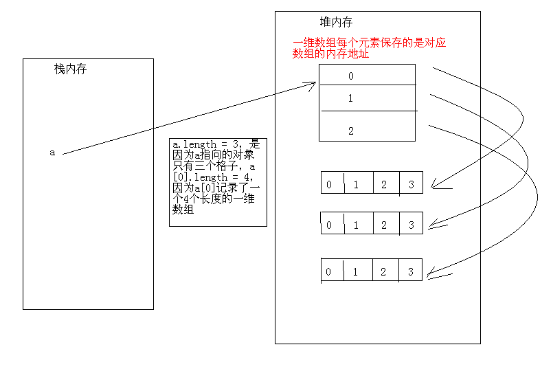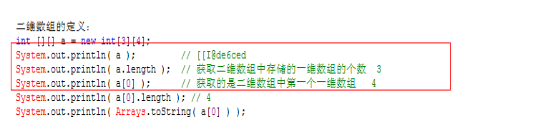new一个数据结构_数据结构严蔚敏为什么用new - CSDN

• 2019.2.19 1.考虑以下二分查找的代码： #include &amp;amp;amp;lt;stdio.h&amp;amp;amp;gt; int bsearch(int array[], int n, int v) { int left, right, middle; left = 0, right = n - ... middle = lef...
2019.2.19
1.考虑以下二分查找的代码：
#include <stdio.h>
int bsearch(int array[], int n, int v)
{
int left, right, middle;
left = 0, right = n - 1;
while (left <= right) {
middle = left + (right - left) / 2;
if (array[middle] > v ) {
right = middle;
} else if (array[middle] < v) {
left = middle;
} else {
return middle;
}
}
return -1;
}

对于输入array为：{2, 6, 8, 10, 13, 25, 36, 45, 53, 76, 88, 100, 127}， n = 13, v = 127时,
运行bsearch函数，while循环调用的次数为____。

1
3
4
5
6
无数次

m的取值为6,9,10,11,11,11,11所以会无限次循环。下面是正确的二分查找

public static int Method(int[] nums, int low, int high, int target)
{
while (low <= high)
{
int middle = (low + high) / 2;
if (target == nums[middle])
{
return middle;
}
else if (target > nums[middle])
{
low = middle + 1;
}
else if (target < nums[middle])
{
high = middle - 1;
}
}
return -1;
}

2.对N个元素的表做顺序查找时，若查找每个元素的概率相同，则平均查找长度为(   )

（N+1）/2
N/2
N
[（1+N）*N ]/2

第一个数的比较次数为1，第二个数的比较次数为2。。。以此类推第N个数的比较次数为N，所以总的比较次数为1+2+…+N=N(N+1)/2,平均比较次数为(N+1)/2,也即平均查找长度。

3.对有18个元素的有序表r[0…17],进行二分查找，则查找r的比较序列下标为（）

0、1、2
8、4、1、2
8、3
8、3、1、2

第一次,下标范围0–17       比较序列r
第二次,下标范围0–7         比较序列r

4.对于关键字序列（16,10,20,12,18,7,14,13,5,19），不可能构成其二叉排序树中一条查找路径的序列是（     ）

16,10,7,5
16,20,18,19
16,10,7,12,14
16,10,12,14

二叉排序树或者是一棵空树，或者是具有下列性质的二叉树：
（1）若左子树不空，则左子树上所有结点的值均小于或等于它的根结点的值；
（2）若右子树不空，则右子树上所有结点的值均大于或等于它的根结点的值；
（3）左、右子树也分别为二叉排序树；

5.设顺序线性表的长度为30，分成5块，每块6个元素，如果采用分块查找并且索引表和块内均采用顺序查找，则其平均查找长度为(     )。

6
11
5
6.5

分块查找会分两部分进行,第一步先进行索引表查找判断其在那个字表中,第二步然后进行在字表中的查找
索引表有5个元素 所以平均查找长度为:(1+5)/2=3
字表中有6个元素,所以平均查找长度为:(1+6)/2=3.5
所以总的平均查找长度为3+3.5=6.5

6.执行()操作时,需要使用队列做辅助存储空间

查找哈希(Hash)表
广度优先搜索网
前序(根)遍历二叉树
深度优先搜索网

深度优先搜索要借助栈；
广度优先搜索要借助队列；

7.具有12个关键字的有序表,折半查找的平均查找长度()

3.1
4
2.5
5

具体查找如下图所示：
查找一次：6.
查找两次：3、10
查找三次：1、4、8、11
查找四次：2、5、7、9、128.顺序表查找指的是在顺序存储结构上进行查找。（ ）

正确
错误

折半查找最坏的情况下查找log(n)+1次，而二叉查找树最坏的情况是查找n次。(退化为单链表)

9.请问对一个排好序的数组进行查找，用平均时间复杂度最小的算法，时间复杂度为（）

O(n)
O(lgn)
O(nlgn)
O(1)10.折半查找与二元查找树的时间性能在最坏的情况下是相同的()

对
错

二叉查找树（英语：Binary Search Tree），也称为二叉搜索树、有序二叉树（ordered binary tree）或排序二叉树
二叉查找树数最坏的情况下是链表的形式。
折半查找最坏的情况是logN+1

11.KMP算法下，长为n的字符串中匹配长度为m的子串的复杂度为（）

O（N）
O（M+N）
O（M+LOGM）
O（N+LOGM）

未解决！！！！！！

12.在一个有8个int数据的数组中，随机给出数组的数据，找出最大和第二大元素一定需要进行（）次比较：

8
9
10
1113. 既希望较快的查找又便于线性表动态变化的查找方法是()

顺序查找
折半查找
分块查找
哈希法查找

索引顺序查找又称为分块查找
顺序查找，算法简单，时间复杂度O(n);
折半查找，要求有序，O(log n);
二叉搜索树，动态，查找性能取决于二叉树的形状，——》二叉平衡树，越平衡，时间复杂度越接近于O(log n); 哈希表：特殊的应用，查找一步到位

2019.2.20
1.设有序顺序表中有n个数据元素，则利用二分查找法查找数据元素X的最多比较次数不超过（）。

log2n+1
log2n-1
log2n
log2(n+1)

平衡二叉树:一棵空树或它的左右两个子树的高度差的绝对值不超过1，并且左右两个子树都是一棵平衡二叉树,查找时间的时间复杂度为O(log2n)。
二叉查找树（Binary Search Tree），或者是一棵空树，或者是具有下列性质的二叉树：若它的左子树不空，则左子树上所有结点的值均小于它的根结点的值；若它的右子树不空，则右子树上所有结点的值均大于它的根结点的值；它的左、右子树也分别为二叉排序树。

设散列表的长度为10，散列函数H(n)=n mod 7，初始关键字序列为 (33，24，8，17，21，10)，用链地址法作为解决冲突的方法，平均查找长度是（）

1
1.5
2
2.5

链地址法，将所有具有相同哈希地址的而不同关键字的数据元素连接到同一个单链表中
33%7=5 24%7=3 8%7=1 17%7=3 放在24后面（24->17）21%7=0 10%7=3放在17后面（24->17->10）
（1+1+1+2+1+3）/6=1.5

从n个数里面找最大的两个数理论最少需要比较

2logn
2 logn -1
n+ logn -2
2n-3

所有次数控制着，最终冠军，每一层的控制着亚军。
类似比赛晋级，两两配对比较，赢的再两两配对，最后得到冠军(最大的数)，可以看成是一棵二叉树，以4人为例：
0
0          2
0    1    2     3
可以看出，找出最大的数比较次数是n-1。然后第二大的数肯定是跟冠军比较过的数字，那么很明显每一层都有一个，所以有logn-1次比较。

所以总共是n+logn-2次比较
4.下面哪一方法可以判断出一个有向图是否有环(回路)( )

深度优先遍历
拓扑排序
Dijkstra求最短路径
求关键路径

绝对能判断有向图是否有环的是：
1.DFS
2.拓扑排序
3.最短路径是允许有环的！C肯定不选。
4.D可选可不选。
关键路径能不能判断一个图有环还存在一些争议。关键路径本身虽然不允许有环，但求关键路径的算法本身无法判断是否有环，判断是否有环的是关键路径的第一步——拓扑排序。所以这个问题的答案主要是看你从哪个角度出发看问题。

若长度为n的线性表采用顺序存储结构,在其第i个位置插入一个新元素的算法的时间复杂度为()(1<=i<=n+1)

O(0)
O(1)
O(n)
O(n2)

6.有 1000 个无序的整数，希望使用最快的方式找出前 50 个最大的，最佳的选择是（ ）

冒泡排序
基数排序
堆排序
快速排序

T(n)=O(f(n))中，函数O（）的正确含义为（）

T(n)为f（n）的函数
T（n）为n的函数
存在足够大的正整数M，使得T（n）≤M×f（n）

8.线性表(a1,a2,…,an)以链接方式存储时,访问第i位置元素的时间复杂性为()

O(i)
O(1)
O(n)
O(i-1)

void recursive(int n, int m, int o)
{
if (n <= 0)
{
printf("%d,%d\n", m, o);
}
else
{
recursive(n - 1, m + 1, o);
recursive(n - 1, m, o + 1);
}
}


以上函数的时间复杂度（）

O(nmo)
O(n2*m2)
O(2^n)
O(n!)

下面是一段求最大值的程序，其中 datalist 是数据表， n 是 datalist 的长度

int GetMax(int n,int datalist[])
{
int k=0;
for(int j=1;j<n;j++)
if(datalist[j]>datalist[k])
k=j;
return k;
}

请问该程序段的 McCabe 环路复杂性为多少？（）

2
3
4
5

以下描述错误的是：

KMP算法的时间复杂度是O(n)
最长路径问题有多项式时间解
最大流问题和最小割问题是等价的
PageRank算法总是会收敛

算法的时间复杂度取决于（）

待处理数据的状态
处理器的速度
问题的规模
程序所占空间


展开全文数据结构
• 定义一个数据结构，下面的操作以这个为蓝本 public class EarthNodeInfo { public EarthNodeInfo() { } public int X { get; set; } public int Y { get; set; } public int Z { get; set;
记录一下,免的后面忘了
定义一个数据结构，下面的操作以这个为蓝本


public class EarthNodeInfo
{

public EarthNodeInfo()
{

}

public int X { get; set; }

public int Y { get; set; }

public int Z { get; set; }

public string GroupName { get; set; }

public string Ico { get; set; }

public int ID { get; set; }

public void SetData(int id,string groupName)
{
this.ID = id;
this.GroupName = groupName;

}

public override string ToString()
{
return $"GroupName: {GroupName} ID: {ID} X: {X} Y: {Y} Z: {Z}"; } }  主要的测试过程  List<string> NameList = new List<string>() { @"道德经", @"抱朴子", @"易经", @"天地大同", @"黑白无双", @"日月神教", @"风花雪月" }; int nameLenght = NameList.Count - 1; List<EarthNodeInfo> EarthLists = new List<EarthNodeInfo>(); List<EarthNodeInfo> EarthLists2 = new List<EarthNodeInfo>(); ///生产数据. Random rm = new Random(); for(int i=0;i<10;i++) { int id = rm.Next(0, 1000); EarthNodeInfo temp = new EarthNodeInfo() { ID = id, GroupName =$"{NameList[rm.Next(0, nameLenght)]}",
X=rm.Next(10,12),Y =rm.Next(0,300),Z=rm.Next(20,500)};
}

for (int i = 0; i < 20; i++)
{
int id = rm.Next(0, 1000);
EarthNodeInfo temp = new EarthNodeInfo()
{
ID = id,
GroupName = $"{NameList[rm.Next(0, nameLenght)]}", X = rm.Next(10, 20), Y = rm.Next(0, 300), Z = rm.Next(20, 500) }; EarthLists2.Add(temp); } //查询ID>5的数据,并输出. var queryList = from m in EarthLists where m.ID > 5 select m; PrintOutData<EarthNodeInfo>(queryList); //查询数据并输出(排序之后) var queryOrderByList = from m in EarthLists where m.ID > 5 orderby m.X descending,m.Y,m.Z select m; PrintOutData<EarthNodeInfo>(queryOrderByList); //方式二: var queryOrderByList_Method = EarthLists.Where(m=>m.ID>5).OrderBy(m=>m.X).ThenByDescending(m=>m.Y).ThenBy(m=>m.Z); PrintOutData<EarthNodeInfo>(queryOrderByList_Method); //从EarthList 和 EarthList2两张表中,查询指定条件的结果.(重新new 一个匿名类.) var queryJoinOnList = from m in EarthLists join m2 in EarthLists2 on m.X equals m2.X where m2.Y > 100 select new { X1 = m.X,X2=m.X ,GroupName = m.GroupName,K=1 }; Console.WriteLine(@"---------------两张表联合,并提取指定数据--------------"); foreach (var v in queryJoinOnList) { Console.WriteLine($"{v.X1}  {v.X2} {v.GroupName}  {v.K}");//输出按照要求排序的键值 age
}

//分组查询(More Key Values);
var queryGroupByList = from Item in EarthLists
group Item by new { Item.GroupName,Item.ID } into Group2
orderby Group2.Count()
select new { className = Group2.Key, count = Group2.Count() };

Console.WriteLine(@"---------------原始数据--------------");
foreach (var v in EarthLists)
{
Console.WriteLine($"{v.ToString()}");//输出按照要求排序的键值 age } Console.WriteLine(@"---------------分组--------------"); foreach (var v in queryGroupByList) { Console.WriteLine($"{v.className}   数量: {v.count}");//输出按照要求排序的键值 age
}



展开全文• 用带表头的链表存放输入的数据，每读入一个数，按升序顺序插入到链表中，链表中允许两个结点有相同值。链表的头结点存放链表后面的结点个数，初始化时就生成头结点（初值为0）。 在上述带表头的链表中删除第i个结点...
实验内容：

（一）单链表的定义及基本操作

用带表头的链表存放输入的数据，每读入一个数，按升序顺序插入到链表中，链表中允许两个结点有相同值。链表的头结点存放链表后面的结点个数，初始化时就生成头结点（初值为0）。
在上述带表头的链表中删除第i个结点或删除数值为item的结点。
（3）链表翻转是把数据逆序（变成降序），注意，头结点不动。翻转后要再翻转一次，恢复升序后才能插入新元素，否则会出错。

（4）设A与B分别为两个带有头结点的有序循环链表（所谓有序是指链接点按数据域值大小链接，本题不妨设按数据域值从小到大排列），list1和list2分别为指向两个链表的指针。请写出并在计算机上实现将这两个链表合并为一个带头结点的有序循环链表的算法。

（二）链式堆栈的定义及基本操作

（5）先定义堆栈的几个基本操作，再设计一主函数利用堆栈的操作完成以下功能：假设一个算术表达式中可以包含三种括号：()[]{}，且这三种括号可以按任意次序嵌套使用（如：...[...{...}...[...]...]...(...)）。编写判别给定表达式中所含括号是否正确配对出现的算法，已知表达式已存入数据元素为字符的单链表中。

（三）链式队列的定义及基本操作

（6）先定义队列的几个基本操作，再设计一主函数利用队列的操作完成以下功能：键盘输入的字符可以临时存入键盘的缓冲区中。为了充分利用缓冲区的空间，往往将缓冲区设计成链式循环队列的结构，并为循环队列结构的缓冲区设置一个队首指针和一个队尾指针。每输入一个字符到缓冲区中，就将尾指针后移，链入缓冲区的循环队列之中；每输出一个字符号，就将队头指针前移，将它从缓冲队列中删除。假设有两个进程同时存在于一个应用程序中，第一个进程连续在屏幕上显示字符“X”，第二个进程不断检查键盘上是否有输入，若有则读入用户键入的字符，将其保存到键盘缓冲区中。

#include <iostream>
#include <conio.h>
using namespace std;
#define MAXQSIZE 100
typedef struct QNode
{
char data;
struct QNode *next;
}QNode,*QueuePtr;
typedef struct
{
QueuePtr front;
QueuePtr rear;
{
Q.front=Q.rear=new QNode;
Q.front->next=NULL;
}
{
QueuePtr p=new QNode;
p->data=e;
p->next=NULL;
Q.rear->next=p;
Q.rear=p;
}
{
if(Q.front==Q.rear) return 0;
QueuePtr p=Q.front->next;
e=p->data;
Q.front->next=p->next;
if(Q.rear==p) Q.rear=Q.front;
delete p;
return 1;
}
int main()
{
InitQueue(Q);
while(1)
{
cout<<'X';
if(kbhit())
{
char ch=getch();
if(ch=='#') break;
EnQueue(Q,ch);
}
}
char e;
while(DeQueue(Q,e))
{
cout<<e;
}
return 0;
}


#include<iostream>
using namespace std;

struct node
{
int val;
node *nex;
node *pre;
node()
{
val=0;
nex=NULL;
pre=NULL;
}
};

{
node *tmp=new node;
tmp->val=val;
if(T->nex==NULL)
{
T->nex=tmp;
return ;
}
node *p=T;
node *s=T->nex;
while(s!=NULL&&s->val<val)
{
p=s;
s=s->nex;
}
if(s==NULL)
{
p->nex=tmp;
}
else
{
p->nex=tmp;
tmp->nex=s;
}
T->val++;
}

void del_id_node(node* &T,int id)
{
if(!T->nex)
{
cout<<"Line is NULL"<<endl;
return ;
}
if(T->val<id)
{
cout<<"no "<<id<<" num\n";
return ;
}

node *p=T;
node *s=T->nex;
int cnt=0;
while(1)
{
cnt++;
if(cnt==id)
{
cout<<"The "<<id<<" node is delete, its val is ";
cout<<s->val<<endl;
p->nex=s->nex;
delete s;
break;
}
p=s;
s=s->nex;
}
node *ne=T->nex;
while(ne!=NULL)
{
cout<<ne->val<<" ";
ne=ne->nex;
}
cout<<endl;
}

void del_val_node(node* &T,int val)
{
node *p=T;
node *s=T->nex;

while(s!=NULL)
{
if(s->val==val)
{
node *t=s;
p->nex=s->nex;
s=s->nex;
delete t;
}
else
{
p=s;
s=s->nex;
}
}
cout<<"The "<<val<<" number is delete"<<endl;
node *ne=T->nex;
while(ne!=NULL)
{
cout<<ne->val<<" ";
ne=ne->nex;
}
cout<<endl;
}

void Reverse(node * &T)
{
if(!T->val)
{
return ;
}
node *p=T;
node *s=T->nex;
while(s->nex!=NULL)
{
s->pre=p;
p=s;
s=s->nex;
}

T->nex=s;
while(p!=T)
{
//cout<<p->val<<" "<<s->val<<endl;
s->nex=p;
s=p;
p=p->pre;
}
s->nex=NULL;
p=NULL;
//cout<<p->val<<endl;
node *ne=T->nex;
while(ne!=NULL)
{
cout<<ne->val<<" ";
ne=ne->nex;
}
cout<<endl;
}

void Join(node* &T3,node* &T2,node* &T1)
{
node *p=T3;
node *p1=T1->nex;
node *p2=T2->nex;
//cout<<p1->val<<" "<<p2->val<<endl;

while(p1!=T1&&p2!=T2)
{
int val1=0;
int val2=0;
val1=p1->val;
val2=p2->val;
//cout<<val1<<" "<<val2<<endl;
if(val1>val2)
{
node *t=new node;
t->val=val2;
p->nex=t;
p=p->nex;
p2=p2->nex;
}
else
{
node *t=new node;
t->val=val1;
p->nex=t;
p=p->nex;
p1=p1->nex;
}
}
if(p1==T1)
{
while(p2!=T2)
{
node *t=new node;
t->val=p2->val;
p2=p2->nex;
p->nex=t;
p=p->nex;
}
}
else
{
while(p1!=T1)
{
node *t=new node;
t->val=p1->val;
p1=p1->nex;
p->nex=t;
p=p->nex;
}
}

cout<<"T1 ans T2 joined is ";
node *ne=T3->nex;
while(ne!=NULL)
{
cout<<ne->val<<" ";
ne=ne->nex;
}
cout<<endl;
}

node* Show(node * &T,int id)
{
node *p=T;
node *ne=T->nex;
while(ne!=NULL)
{
cout<<ne->val<<" ";
p=ne;
ne=ne->nex;
}
cout<<endl;
return p;
}

int n;
int main()
{
//(1)
node *T=new node;
cin>>n;
while(n--)
{
int a;
cin>>a;
}
Show(T,1);

//(2)
del_id_node(T,3);
del_val_node(T,12);

//(3)
Reverse(T);

//(4)循环链表合并
node *T1=new node;
cin>>n;
while(n--)
{
int a;
cin>>a;
}
node *p=Show(T1,1);
p->nex=T1;//指向头指针

node *T2=new node;
cin>>n;
while(n--)
{
int a;
cin>>a;
}
p=Show(T2,2);
p->nex=T2;

node *T3=new node;

Join(T3,T2,T1);

return 0;
}


#include <iostream>
#include <cstring>
using namespace std;

#define MAXSIZE 100
#define OVERFLOW 0
#define OK 1
#define ERROR 0
#define NO 0

typedef struct StackNode
{
char data;
struct StackNode *next;

{
S=NULL;
return OK;
};

{
p->data=e;
p->next=S;
S=p;
return OK;
}

{
if(S==NULL) return ERROR;
e=S->data;
S=S->next;
delete p;
return OK;
}

{
if(S!=NULL)
return S->data;
else return ERROR;
}

{
int len=str.length();
//cout<<len<<endl;
for(int i=0;i<len;i++)
{
if(str[i]!='('&&str[i]!=')'&&str[i]!='['&&str[i]!=']'&&str[i]!='{'&&str[i]!='}') continue;

if(S==NULL)
Push(S,str[i]);
else
{
//cout<<GetTop(S)<<" ";
if(GetTop(S)=='('&&str[i]==')')
{
char e;
Pop(S,e);
}
else if(GetTop(S)=='{'&&str[i]=='}')
{
char e;
Pop(S,e);
}
else if(GetTop(S)=='['&&str[i]==']')
{
char e;
Pop(S,e);
}
else
{
Push(S,str[i]);
}
}
}
if(!S)
return OK;
else return NO;
}

int main()
{
string str;
//freopen("input.txt","r",stdin);
while(cin>>str)
{
cout<<str<<endl;
InitStack(S);
bool f=Check(S,str);
if(f) cout<<"YES"<<endl;
else cout<<"NO"<<endl;
}
return 0;
}

typedef struct QNode
{
char data;
struct QNode *next;
}QNode,*QueuePtr;
typedef struct
{
QueuePtr front;
QueuePtr rear;
{
Q.front=Q.rear=new QNode;
Q.front->next=NULL;
}
{
QueuePtr p=new QNode;
p->data=e;
p->next=NULL;
Q.rear->next=p;
Q.rear=p;
}
{
if(Q.front==Q.rear) return 0;
QueuePtr p=Q.front->next;
e=p->data;
Q.front->next=p->next;
if(Q.rear==p) Q.rear=Q.front;
delete p;
return 1;
}
int main()
{
InitQueue(Q);
while(1)
{
cout<<'X';
if(kbhit())
{
char ch=getch();
if(ch=='#') break;
EnQueue(Q,ch);
}
}
char e;
while(DeQueue(Q,e))
{
cout<<e;
}
return 0;
}


展开全文数据结构实验
•  数组其实就是一个可以装任意类型数据的集合，可以看作为一个容器。虽然可以装任意类型的数据，但是数组只要定义之后，数组里面就是只能装同一数据类型的元素。  数组是属于引用数据类型（数组名中存储的是内存首...
一维数组

1.数组的概念

数组其实就是一个可以装任意类型数据的集合，可以看作为一个容器。虽然可以装任意类型的数据，但是数组只要定义之后，数组里面就是只能装同一数据类型的元素。

数组是属于引用数据类型（数组名中存储的是内存首地址）

数组在内存中是一个连续的存储空间。

数组本身只有length属性（length获取数组中能存储的数据个数）

2.数组的好处

可以给装进来的数据自动排序以及编号。

直接通过下标进行定位的。

3.数组的格式

格式1：元素类型【】 数组名 = new 元素类型【】；

int[] a = new int[];(推荐)

int a[] = new int[];(不推荐)

格式2：元素类型【】 数组名 = {元素1，元素2，元素3......};

int[] a = {1,2,3,4};

注意：给数组分配空间时，必须要指定存储的个数大小来确定数组的大小。数组的大小创建后不可修改。

4.数组清零（便利赋值）

for(int j = 0;j<arg.length-1;j++){

arg[j]=null;

}

5.一维数组的内存分析二维数组

可以说java中其实并没有二维数组和三维数组等等，都是由一维数组扩展而来。所以说实际上也是一维数组。

1. 数组定义：

数组类型[][] 数组名 = new 数组类型[一维数组的个数][每一个一维数组中元素的个数];

例：int[][] a = new int;

int[][] b = {{123},{2134},{45114}};

在内存中如下图，栈中对象a有地址指向一维数组的元素，一维数组的元素保存的地址再指向对应一维数组。2.二维数组清零

和一维数组清零类似，但外面要套一个for循环

3.二维数组Arrays的使用

遍历： toString()    将数组的元素以字符串的形式返回

排序： sort()        将数组按照升序排列

查找： binarySearch()在指定数组中查找指定元素，返回元素的索引，如果没有找到返回（-插入点-1） 注意：使用查找的功能的时候，数组一定要先排序。参考：https://blog.csdn.net/oguro/article/details/52971487
展开全文• 在做项目数据同步开发的时候，我碰到一个很奇怪的显现。我首先从数据库获取数据集DataSet然后用Model把数据封装，然后放到List中，最后我遍历List时发现，它里面只放了最后一条数据封装的model.我把代码贴出来如下: ...
• 在学习数据结构与算法的时候，T *p = new T[n]的算法复杂度为Θ(n)，而int *p = new int[n]的算法复杂度为Θ(1)。 C++ primer的动态内存的讲解没有关于这一点的解释，自己测试了一下：#include using namespace std...c++ struct 算法复杂度
• ## 数据结构：八大数据结构分类

万次阅读 多人点赞 2018-09-05 18:23:28
数据结构是指相互之间存在着种或多种关系的数据元素的集合和该集合中数据元素之间的关系组成 。 常用的数据结构有：数组，栈，链表，队列，树，图，堆，散列表等，如图所示： 每数据结构都有着独特的数据...数据结构
• ## 数据结构基础概念篇

万次阅读 多人点赞 2017-11-14 13:44:24
数据结构一些概念 数据结构就是研究数据的逻辑结构和物理结构以及它们之间相互关系，并对这种结构定义相应的运算，而且确保经过这些运算后所得到的新结构仍然是原来的结构类型。数据：所有能被输入到计算机中，且能...数据结构
• ## JAVA常用数据结构

万次阅读 多人点赞 2018-07-30 14:12:59
背景 ...本文将对这些常用的JAVA中的数据结构进行一个简单的介绍。 JAVA中的数据结构简述 JAVA中常用的数据结构主要有这样几种分类： 1. List：可存储相同的值（确切讲是a.equals(b)时，二者...JAVA 数据结构
• ## 数据结构——小白入门篇

万次阅读 多人点赞 2018-07-14 19:35:07
****在计算机界有这样一个万能公式：数据结构 + 算法 = 程序。****在如今这计算机引领风骚的时代，不学数据结构，你凭什么想要做时代的弄潮儿；所以我毅然决然的提前自学了数据结构。 ***学习数据结构前的我是这样...数据结构 数据结构C++实现
• ... 数据结构（C语言版 第2版）课后习题答案 严蔚敏 等 编著，仅供参考，还是自己认真做了再看 ...（1）在数据结构中，从逻辑上可以把数据结构分成（ C ）。 A．动态结构和静态结构 B．紧凑...数据结构
• ## java 中几种常用数据结构

万次阅读 多人点赞 2016-07-25 19:26:46
java中有几种常用的数据结构，主要分为Collection和map两主要接口（接口只提供方法，并不提供实现），而程序中最终使用的数据结构是继承自这些接口的数据结构类。其主要的关系（继承关系）有： （----详细参见java...数据结构 java
• ## 为什么要学数据结构？

万次阅读 多人点赞 2019-11-19 13:54:58
一、前言 在可视化化程序设计的今天，借助于...1) 能够熟练地选择和设计各种数据结构和算法 2) 至少要能够熟练地掌握一门程序设计语言 3) 熟知所涉及的相关应用领域的知识 其中，后两个条件比较容易实现，而第一个...数据结构 算法 线性结构 树结构java 数据结构 算法 stack
• ## 数据结构基础知识核心归纳(一)

万次阅读 多人点赞 2017-09-10 00:12:02
堆是种树状的数据结构。一般由程序员分配释放，存放由new创建的对象和数组(C中是由malloc分配和free释放)，JVM不定时查看这对象，如果没有引用指向这对象就回收.1)优点：可动态分配内存大小，生成周期不必事先...数据结构 Android面试 笔试
• 算法是为求解一个问题需要遵循的、被清楚指定的简单指令的集合。下面是自己整理的常用数据结构与算法相关内容，如有错误，欢迎指出。 为了便于描述，文中涉及到的代码部分都是用Java语言编写的，其实Java本身对...常见数据结构与算法
• 程序设计主要是数据结构+算法，而数据结构在面向对象思维里是“容器”的意思，数据结构主要负责数据的添加，删除，修改，查找及对数据的其他操作。编程里面对着不同问题场景，选择哪种数据结构进行操作就非常重要。...数据结构 java
• ## 超清晰-数据结构之线性表

万次阅读 多人点赞 2020-04-03 21:01:14
鸟哥说，坚持学习基础才能有出人头地的一天。不能只专注于练武功了，内功也得练。 本篇文章是讲数据结构的第一篇，跟着书好好再过一篇基础。...（1）存在唯一的一个被称作“第一个”的数据元素； （2）...顺序表 链表 单链表
• ## 数据结构与算法-单链表篇

千次阅读 多人点赞 2018-07-31 15:49:32
也是非常基础的数据结构，往往简单的东西越需要扎实，大多数的情况是眼高手低觉得很简单差不多，写代码就出错，运行就崩溃，完成链表这一数据结构主要在于对指针的使用。  链表是种线性的数据结构，相比...数据结构与算法 单链表
• ## Java数据结构与算法解析(一)——表

万次阅读 多人点赞 2020-09-24 14:34:38
线性表是一个线性结构，它是一个含有n≥0个结点的有限序列，对于其中的结点，有且仅有一个开始结点没有前驱但有一个后继结点，有且仅有一个终端结点没有后继但有一个前驱结点，其它的结点都有且仅有一个前驱和一个...java 数据结构 算法 ArrayList
...